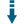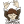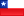# PHP Mini Language: Execute string of a simple language conditions

 Recommend this page to a friend!ReTweetDownload .zip
 InfoDocumentationView files (17)Download .zip Reputation Support forum Blog Links
 Last Updated Ratings Unique User Downloads Download Rankings 2020-03-28 (2 months ago)Not yet rated by the users Total: 93 All time: 9,426 This week: 362Version License PHP version Categories minilang 1.0.1 GNU Lesser Genera... 5 PHP 5, Language, Parsers
Description Author

This package can execute string of a simple language conditions.

It can take a text string with an expression that defines conditions to be evaluated by a the package that can return the result of the evaluation.

The expression may contain variables with values to be passed at run time and can be evaluated with boolean operators.

It may also also combine different expressions that can assign variable values depending on conditions, so the new values may affect the results of the evaluation of other expressions.

Innovation AwardDecember 2019 Number 6 Some applications need to evaluate conditions that are only defined at run time. Therefore the conditions cannot be evaluate with code that was previously written. This package provides a solution to evaluate dynamic conditions by parsing one or more text scripts that contain condition expressions. Then they can combine the evaluation of the condition results into a final result according to the needs of the application. Manuel LemosPerformance Level
Name: Classes: Jorge Castro `` 17 packages by Jorge CastroChile 44 1752 5 in Chile361 in ChileInnovation awardNominee: 8x

Winner: 1x

Details

# MiniLang

A mini script language for PHP. It does three simple tasks.

1. (optional) It set some initial values (INIT).
2. It evaluates a logic expression (WHERE).
3. And if the expression (logic) is true then it executes the SET expression (SET).

For example :

> when var1>5 and var2>20 then var3=20

> init var5=20 when var1>5 and var2>20 then var3=var5[]() []() []() []() []()

## Why we need a mini script?

Sometimes we need to execute arbitrary code in the basis of "if some value equals to, then we set or execute another code."

In PHP, we could do the next code.

``````if(\$condition) {
\$variable=1;
}
``````

However, this code is executed at runtime. What if we need to execute this code at some specific point?.

We could do the next code:

``````\$script='if(\$condition) {
\$variable=1;
}';

// and later..
eval(\$script);
``````

This solution works (and it only executes if we call the command eval). But it is verbose, prone to error and it's dangerous.

Our library does the same but safe and clean.

``````\$mini->separate("when condition then variable=1");
``````

## Getting started

Installing it using composer:

> composer requires eftec/minilang

Creating a new project

``````use eftec\minilang\MiniLang;
include "../lib/MiniLang.php"; // or the right path to MiniLang.php
\$mini=new MiniLang();
\$mini->separate("when field1=1 then field2=2"); // we set the logic of the language but we are not executed it yet.
\$mini->separate("when field1=2 then field2=4"); // we set more logic.
\$result=['field1'=>1,'field2'=>0]; // used for variables.
\$callback=new stdClass(); // used for callbacks if any
\$mini->evalAllLogic(\$callback,\$result);
var_dump(\$result);
``````

## Methods

### Constructor

> __construct(&\$caller,&\$dict,array \$specialCom=[],\$areaName=[],\$serviceClass=null)

• object \$caller Indicates the object with the callbacks
• array \$dict Dictionary with initial values
• array \$specialCom Special commands. it calls a function of the caller.
• array \$areaName It marks special areas that could be called as "<namearea> somevalue." *null|object \$serviceClass A service class. By default, it uses the \$caller.

### reset()

It reset the previous definitions but the variables, service and areas.

### setCaller(&\$caller)

Set a caller object.

### setDict(&\$dict)

Set a dictionary with the variables used by the system.

### function separate(\$miniScript)

It sends an expression to the MiniLang, and it is decomposed in its parts. The script is not executed but parsed.

### evalLogic(\$index)

It evaluates a logic. It returns true or false.

• \$index is the number of logic added by separate()

### evalAllLogic(\$stopOnFound = true, \$start = false)

• bool \$stopOnFound exit if some evaluation matches
• bool \$start if true then it always evaluates the "init" expression.

### evalSet(\$idx = 0, \$position = 'set')

It sets a value or values. It does not consider if WHERE is true or not.

• int \$idx number of expression
• string \$position =['set','init'][\$i] It could be set or init

## Definition

### Sintaxis.

The syntaxis of the code is separated into four parts. INIT, WHERE (or when), SET (or THEN) and ELSE.

Example:

``````\$mini->separate("when field1=1 then field2=2");
``````

It says if field1=1 then we set field2 as 2.

### Variables

A variable is defined by `varname`

Example: examples/examplevariable.php

``````\$mini=new MiniLang();
\$mini->separate("when field1>0 then field2=3"); // we prepare the language
\$variables=['field1'=>1]; // we define regular variables
\$callback=new stdClass();
\$mini->evalAllLogic(\$callback,\$variables); // we set the variables and run the languageand run the language
var_dump(\$variables); // field1=1, field2=3
``````

### Variables defined by a PHP Object

A variable could host a PHP object, and it is possible to call and to access the fields inside it.

`varname.field`

• If the field exists, then it uses it.
• If the field doesn't exist, then it uses a method of the object CALLER.
• If the method of the CALLER doesn't exist then it tries to use the method of the service class
• If the method of the service class doesn't exist then it tries to use the inner method of the class MiniLang (with a prefix _). Example function _param()
• Finally, if everything fails then it triggers an error.

Example of code examples/examplevariable2.php

``````class MyModel {
var \$id=1;
var \$value="";
public function __construct(\$id=0, \$value="")
{
\$this->id = \$id;
\$this->value = \$value;
}
}
class ClassCaller {
public function Processcaller(\$arg) {
echo "Caller: setting the variable {\$arg->id}<br>";
}
}
class ClassService {
public function ProcessService(\$arg) {
echo "Service: setting the variable {\$arg->id}<br>";
}
}
\$mini=new MiniLang([],[],new ClassService());
\$mini->separate("when field1.id>0 then
field2.value=3
and field3.processcaller
and processcaller(field3)
and processservice(field3)"); // we prepare the language
\$variables=['field1'=>new MyModel(1,"hi")
,'field2'=>new MyModel(2,'')
,'field3'=>new MyModel(3,'')]; // we define regular variables
\$callback=new ClassCaller();
\$mini->evalAllLogic(\$callback,\$variables,false); // we set the variables and run the languageand run the language
var_dump(\$variables);
``````

• field2.value references the field "value" (MyModel)
• field3.processcaller references the method ClassCaller::processcaller()
• processcaller(field3) does the same than field3.processcaller
• processservice(field3) calls the method ClassService::processservice()

### Variables defined by a PHP array

A variable could hold an associative/index array, and it is possible to read and to access the elements inside it.

``````vararray.associndex // vararray['associindex']
vararray.4 // vararray
vararray.param('a.b.c') // vararray['a']['b']['c']
param(vararray,'a.b.c') // vararray['a']['b']['c']
``````

• If the element exists, then it uses it.
• If the element doesn't exist, then it uses a method of the caller.
• If the method of the caller doesn't exist then it tries to use the method of the service class
• Finally, if everything fails then it triggers an error.

Example of code examples/examplevariable_arr.php

``````class ClassCaller {
public function Processcaller(\$arg) {
echo "Caller: setting the variable {\$arg['id']}<br>";
}
}
class ClassService {
public function ProcessService(\$arg) {
echo "Service: setting the variable {\$arg['id']}<br>";
}
}

\$mini=new MiniLang([],[],new ClassService());
\$mini->separate("when field1.id>0 then
field2.value=3
and field3.processcaller
and processcaller(field3)
and processservice(field3)");

\$variables=['field1'=>['id'=>1,'value'=>3]
,'field2'=>['id'=>2,'value'=>'']
,'field3'=>['id'=>3,'value'=>'']];
\$callback=new ClassCaller();
\$mini->evalAllLogic(\$callback,\$variables,false);
var_dump(\$variables);
``````

• field2.value references the element "value" of the array
• field3.processcaller references the method ClassCaller::processcaller()
• processcaller(field3) does the same than field3.processcaller
• processservice(field3) calls the method ClassService::processservice()

### Global variables

A global variable takes the values of the PHP (\$GLOBAL), so it doesn't need to be defined or set inside the language

A global variable is defined by

`\$globalname`

``````\$globalname.associndex // \$globalname['associindex']
\$globalname.4 // \$globalname
\$globalname.param('a.b.c') // \$globalname['a']['b']['c']
param(\$globalname,'a.b.c') // \$globalname['a']['b']['c']
``````

For example:

`\$globalname=30`

Example Code: examples/exampleglobal.php

``````\$field1=1; // global variable
\$mini=new MiniLang();
\$mini->separate('when \$field1>0 then \$field1=3'); // we prepare the language
\$variables=[]; // local variables
\$callback=new stdClass();
\$mini->evalAllLogic(\$callback,\$variables); // we set the variables and run the languageand run the language
var_dump(\$field1); // returns 3
``````

## Literals

| Type | Example | |------|---------| |Number|20| |string|"hello world", 'hello world'| |stringp|"my name is {{var}}"| |function|namefunction(arg,arg)|

### examples

> set var=20 and var2="hello" and var3="hello {{var}}" and var4=fn()

### Reserved methods

| Reserved word | Explanation | |---------------|------------------------------------------------------------------------------| | null() | null value | | false() | false value | | true() | true value | | on() | 1 | | param(var,'l1.l2.l3') | Separates an array (var) into var['l1']['l2']['l3'] | | off() | 0 | | undef() | -1 (for undefined) | | flip() | (special value). It inverts a value ON<->OFF<br>Used as value=flip() | | now() | returns the current timestamp (integer) | | timer() | returns the current timestamp (integer) | | interval() | returns the interval (in seconds) between the last change and now. It uses the field dateLastChange or method dateLastChange() of the callback class | | fullinterval() | returns the interval (in seconds) between the start of the process and now. It uses the field dateInit or method dateInit() of the callback class |

Example: examples/examplereserved.php

``````\$mini=new MiniLang();
\$mini->separate("when true=true then field1=timer()"); // we prepare the language
\$variables=['field1'=>1]; // we define regular variables
\$callback=new stdClass();
\$mini->evalAllLogic(\$callback,\$variables); // we set the variables and run the language
var_dump(\$variables);
``````

Example Timer: examples/examplereservedtimer.php

``````class ClassWithTimer {
var \$dateLastChange;
public function dateInit() {
return time();
}
public function __construct()
{
\$this->dateLastChange=time();

}
}
\$mini=new MiniLang();
\$mini->separate("when true=true then field1=interval() and field2=fullinterval()"); // we prepare the language
\$variables=['field1'=>0,'field2'=>0]; // we define regular variables
\$callback=new ClassWithTimer();
\$mini->evalAllLogic(\$callback,\$variables); // we set the variables and run the language
var_dump(\$variables);
``````

## init

This part of the expression allows setting a value. This expression is usually optional, and it could be omitted.

> It is similar to SET, but it is executed before WHERE and no matter if WHERE is valid or not.

> init counter=20 where variable1=20 set variable+counter

• it set the counter to 20.
• And compares: variable1 is equaled to 20
• If yes, then increases a variable by counter

### Code:

``````\$mini->separate("init tmp=50 when condition=1 then field1+10"); // set tmp to 50. If condition is 1 then it increases the field1 by 10.
``````

## where

This part of the expression adds a condition to the statement.

We can also use "when."

> where expression

or

> when expression

It's possible to compare more than a condition at the same time by separating by "and" or "or."

> where v1=10 and v2=20 or v3<50

### Example

> where variable1=20 and \$variable2=variable3 or function(20)=40

> where \$field=20 and field2<>40 or field3=40 // sql syntax

> where \$field==20 && field2!=40 || field3=+40 // PHP syntax

> where 1 // it always true

### Expressions allowed

• equals: = and ==
• not equals: <> and !=
• less than: <
• less or equal than: <=
• greater than: >
• greater or equal than: >=
• contain a text: contain
• and logic: "and" and &&
• or logic: "or" and ||

## set

This part of the expression allows setting the value of a variable. It is possible to set more than one variable at the same time by separating by "," or "and."

We can also use "then."

> set expression

or

> then expression

> SET is only executed if WHERE is valid

It is also possible to set a function. However, the value could not be set (but the function is executed)

### Expressions allowed

We could set a variable using the next expressions:

• variable=20
• variable=anothervariable
• variable=20+30
• variable=40*50+30
• variable+30 // it increases the variable by 30
• variable-30 // it decreases the variable by -30
• variable=flip() // it flips the value 0->1 or 1->0

This library does not allow complex instruction such as

• variable=20+30*(20+30) // is not allowed.

### Example:

> set variable1=20 and \$variable2=variable3 and function(20)=40

### Code:

``````\$mini->separate("when condition=1 then field1+10"); // if condition is 1 then it increases the field1 by 10.
``````

## else

This optional part of the expression allows setting the value of a variable. It is possible to set more than one variable at the same time by separating by "," or "and".

> This code is only evaluated if "where" returns false of if ELSE is called manually.

### Example

> else variable1=20 and \$variable2=variable3 and function(20)=40

### Benchmark

examples/examplebenchmark.php

We call the some operations 1000 times.

#### (reset+separate+evalAllLogic) x 1000

• We call the method reset(), separate() and evalAllLogic 1000 times.
• Speed: 0.028973 seconds. Comparison: 46.6% (smaller is better)

#### evalAllLogic x 1000

• We call the method reset() and separate() 1 time
• And we call the method evalAllLogic() 1000 times.
• Speed: 0.002387 seconds. Comparison: 3.84% (smaller is better)

#### (reset+separate2+evalAllLogic2) x 1000

• We call the method reset(), separate2() and evalAllLogic2() 1000 times.
• Speed: 0.06217 seconds. Comparison: 100% (smaller is better). It is the slower.

#### (evalAllLogic2) x 1000

• We call the method reset() and separate2() 1 time
• And we call the method evalAllLogic2() 1000 times.
• Speed: 0.013418 seconds. Comparison: 21.58% (smaller is better)

#### PHP method of class x 1000

• We create a class with the method \$mini->generateClass2(); 1 time
• Then, we call the class (as simple php code) 1000 times.
• Speed: 0.000763 seconds. Comparison: 1.23% (smaller is better) it is the fastest.

## Documentation

Medium-Creating a new scripting language on PHP

## To-do

• Documentation.

## Version

• 2.15 2020-12-01 * fixed the evaluation of 'set variable=function(variable2)' where function is a php function
• 2.14 2019-10-26 * Fixed the method callFunction() when the first argument of the function is an object but the method is defined in the caller or service class
• 2.12 2019-10-21 * New method separate2() and evalAllLogic2() it works with PHP's eval. * New method generateClass2() * separate2() and evalAllLogic2() works by parsing the tokens and converting in native PHP code. * However it is from x2 to x5 slower than evalAllLogic(). * This new motor could work by caching the evaluation in fields \$setTxt,\$whereTxt,\$elseTxt,\$initTxt * See benchmark
• 2.11 2019-10-11 method _param (class). Also, \$a.fn() is allowed.
• 2.10 2019-10-07 method create()
• 2.9 2019-08-28
• * set field.value=20 , where field is an array works. However field.1=20 does not work (the parser considers .1 as a decimal)
• 2.8 2019-08-26
• * if a field (inside where section) is an object. then it is possible to call the method as field.method(arg)
• * Method getDictEntry()
• 2.7 2019-08-04
• * Added the methods serialize() and unserialize().
• * setCaller() argument is not a reference anymore (objects are reference by default)
• 2.6 2019-08-03 Now it allows "else". Example: "where exp then exp else exp"
• 2.5 2019-08-03 Now it allows to reference index of an array (numeric or associative)
• 2.4 2019-08-02 Added more documentation. Now we allow unitary expression.
• 2.3 2019-05-24 Fixed some bug (if the method is not defined)
• 2.0 2019-05-20 Second version. It uses PHP to parse the file.
• 1.15 2019-01-06 If we add (+) two values and they are numeric then we add. Otherwise, it concatenates.
• 1.14 2018-12-26 First open-source version.Files
File Role Descriptionexamples (8 files)lib (1 file)tests (3 files).travis.yml Data Auxiliary datacomposer.json Data Auxiliary dataLICENSE Lic. License textphpunit.xml Data Auxiliary dataREADME.md Doc. DocumentationFiles / examples
File Role Descriptionexample1.php Class Class sourceexamplebenchmark.php Class Class sourceexampleglobal.php Example Example scriptexamplereserved.php Example Example scriptexamplereservedtimer.php Class Class sourceexamplevariable.php Example Example scriptexamplevariable2.php Class Class sourceexamplevariable_arr.php Class Class sourceFiles / lib
File Role DescriptionMiniLang.php Class Class sourceFiles / tests
File Role DescriptionAbstractMiniLang.php Class Class sourceCompilationTest.php Class Class sourceCompilationTest2.php Class Class source

 All time: 9,426 This week: 362Advertise on this siteSite mapNewsletterStatisticsSite tipsPrivacy policyContact
For more information send a message to `info at phpclasses dot org`.